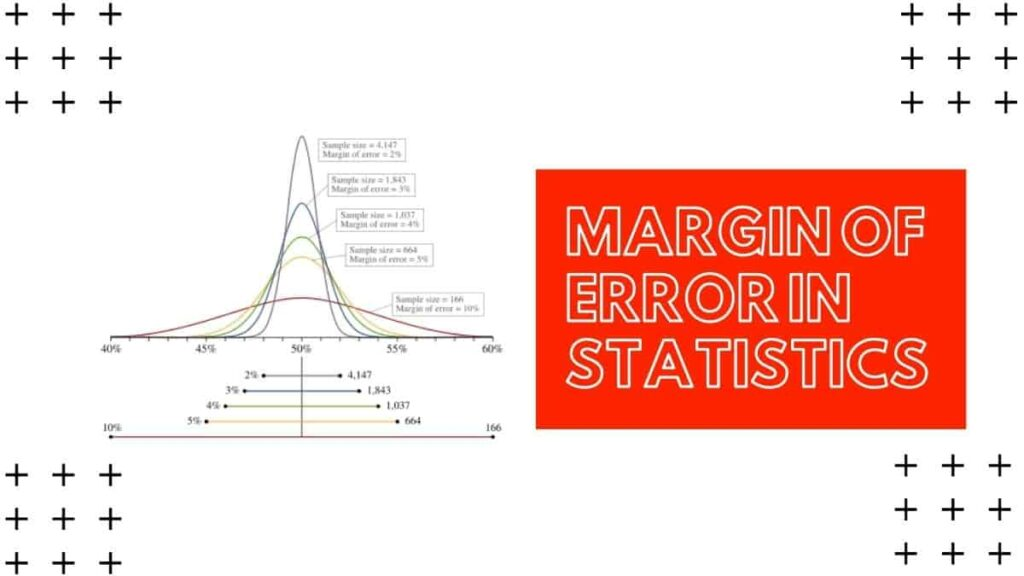# The Definitive Guide on Margin of Error in Statistics

In this article we will give you all the vital information on the “margin of error in statistics” This is very vast to compile in just one small article but still we will give you all the essential information just give complete reading to this piece of compilation.

We will be covering all these questions like what is the margin of error in statistics, How to Calculate Margin of Error, How to Calculate Margin of Error: Steps, Some Relationships, Margin of Error Calculation and some more related information/formulas.

## What is a margin Error?

The range of values below and above the sample statistic in a confidence interval is called the margin of error. We can also define confidence interval as the way to show the uncertainty with a certain static. for Example from a poll result we get that 98% of confidence interval of 4.88 and 5.26. And if the poll is repeat again using the same parameter and technique. Then we will definitely get results in the interval 4.88 to 5.26 98% of the times.

## What is Margin of Error Percentage ?

Margin of error is defined as the difference between true population. And the estimated population from poll result is called “Error percentage of margin”. We can calculate margin of error using these formulas given below :

Margin of error = Product of Critical value and Standard deviation or Margin of error = Product of Critical value and Standard error of the statistic.

## How to calculate the margin of error: Steps

### Step 1

Calculate the critical value.

### Step 2

Calculate the standard deviation or the standard Error.

### Step 3

Calculate the Product of critical value with standard deviation or the standard Error. For example critical value= 1.5 and Standard deviation=0.06 then the margin of error calculation will be ‭0.09‬.

calculate using t-distribution calculator on this site find the t-score and the variance and standard deviation calculator will calculator will find the standard deviation from sample.

## Margin of Error for a Proportion

To calculator proportion we need sample proportion, sample size and z-score

Where   Z is the Z-score.

is the Sample proportion.

n is sample space.

For Example.

A group of people did a survey on 1000 scientists and 380 of thought that climate change was not caused by human pollution. Find the MoE for a 90% confidence interval

### Calculate P-hat

Calculate P-hat(sample proportion) by dividing the no. of people who agreed climate change was not caused by human pollution. It means that they answered according to the statement in this example 38% scientist responded positively.

### Calculate Z-Score

Calculate the z-score that goes with confidence interval. you’ll need to reference this critical values. A 90% confidence interval has a z-score of 1.64.

### Use Margin of Error Formula

Using the formula of the margin of error find the value.

=1.64√[{0.38(0.62)}1000]

=1.64*0.0153=0.0252

### Get the Result

Calculate the percentage of the step 3 and then we get thee value of the margin of error is 2.52%.

## Statistics aren’t accurate Use of margin of Error

• It is a very useful method for estimating any value in this method like Calculations assuming random sampling, Different confidence levels plays an important role and also effect of sample size matters a lot. And as the heading the static are never accurate use of margin of error provide better resolution to the  estimated result from any polls or survey.
• Also this process is easy to estimate as we don’t have to cover the entire size of the things rather we just have to focus on the small sample part of the entire thing and this small sample represents the entire thing. Besides, this way the cost of surveying the entire population.

## Conclusion

Now you might be quite confident about the margin of error in statistics. Next time don’t get into trouble while talking about the margin of error in statistics.

Furthermore, get the best math assignment help from the most reliable math assignment helpers in the world.

## More Question on Margin of Error in Statistics

### What is a margin Error?

The range of values below and above the sample statistic in a confidence interval is called the margin of error.

### What is Margin of Error Percentage ?

Margin of error is defined as the difference between true population. And the estimated population from poll result is called “Error percentage of margin”.